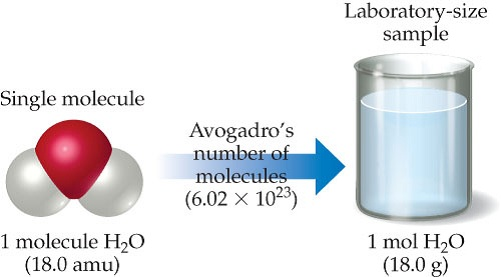# Problem: Comparing the mass of 1 molecule and 1 mol of H2O. Both masses have the same number but different units (atomic mass units and grams). Expressing both masses in grams indicates their huge difference: 1 molecule H2O has a mass of 2.99 10-23 g whereas 1 mol H2O has a mass of 18.0 g.How many H2O molecules are in a 9.00-g sample of water?

###### FREE Expert Solution

For water, H2O

1 mol H2O = 18.0 g H2O
1 mole H2O = 6.022x1023 H2O molecules

92% (75 ratings)###### Problem DetailsComparing the mass of 1 molecule and 1 mol of H2O. Both masses have the same number but different units (atomic mass units and grams). Expressing both masses in grams indicates their huge difference: 1 molecule H2O has a mass of 2.99 10-23 g whereas 1 mol H2O has a mass of 18.0 g.

How many H2O molecules are in a 9.00-g sample of water?

Frequently Asked Questions

What scientific concept do you need to know in order to solve this problem?

Our tutors have indicated that to solve this problem you will need to apply the Mole Concept concept. You can view video lessons to learn Mole Concept. Or if you need more Mole Concept practice, you can also practice Mole Concept practice problems.